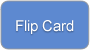# Math QuestionsWhat is Algebra?

1 of
Show both sides

20% Complete (success)

What is Algebra?

### Term

What is Geometry?What is Algebra?

### Term

What is TrigonometrWhat is Algebra?

### Term

What is Calculus?What is Algebra?

### Term

What is Statistics?What is Algebra?Terms Definitions
What is Algebra? Algebra uses both letters and numbers in equations and formula. The letters (commonly x and y) represent unknown quantities. Formula are used to set up equations which can be used to solve for the letter representing the quantity.
What is Geometry?What is Algebra? Geometry involves the relationship of lines and points. Solids and surfaces are also explored as part of this discipline. It can also be used to address higher analogs of dimension beyond circles, squares, and common shapes.
What is TrigonometrWhat is Algebra? Trigonometry deals with triangles. This mathematical discipline addresses the relationship between the angles and sides of the triangle. The function of the angles in any given triangle is also analyzed and considered.
What is Calculus?What is Algebra? Derivatives and integrals of functions are studied in calculus. Finding them and examining their properties are both important. There are two types of this discipline, which are integral and differential calculus. The methods used are based on infinitesimal differences and their specific summation.
What is Statistics?What is Algebra? Statistics involves analyzing numerical data that has been collected in large quantities. The goal is to determine what the information provides in the way of expanding that information to a larger population. By examining a sample, conclusions can be extrapolated to a larger group of individuals.